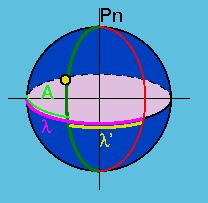Line of Positions

# Lines of Positions

1. The Celestial Sphere
2. The Astronomical Triangle
3. Lines of Position
4. References and Postscript Code

If the resources are not available to solve the astronomical triangle, a method of plotting "lines of position" is a reliable way to pinpoint your location. The geometry of this method is explained below and step by step instructions are given for determining your location.

Step 1: Find the current Estimated Position (EP). If this method is used throughout the voyage, and is performed regularly, then it is not dificult to calculate the estimated position by using d=vt, and performing simple vector manipulations to account for drift due to currents. The EP need only be within a 50 mile radius of the actual position. Plot the EP on a map or chart.

Step 2: Use a sextant to observe the altitude of 2 or more stars. Use an Almanac to make the appropriate corrections. The resulting observed altitude is called Ho.

Step 3: Compute the azimuth A of each star.Figure 7

A = l +/- l'

where l is the estimated latitude, and l' is the latitude of the celestial body at the current time - found with an almanac.

Step 4: Compute the calculated altitude Hc by using the first sight reduction formula (1), the estimated position, the calculated azimuth, and the declination and GHA of the star from the almanac.

Step 5: Calculate the intercept (ITC).Figure 8

ITC = Ho - Hc

Step 6: On the map or chart, draw 'lines of bearing' from the EP to the groundpoint of each star. The groundpoint of the star is the coordinates of the projection of the star onto the earth, and is obtainable in any astronomy almanac.Figure 9

Step 7: Draw "lines of position". Each Line of position must be perpendicular to their line of bearing, and must cross the line of bearing at a distance of ITC from the EP. Your actual location is where the lines of position cross (O on Figure 10 below). If using more than 2 stars, it is unlikely that the Lines of position will all cross in exactly the same spot due to small errors. In this case the method of least squares is used to obtain the best fit, and determine location.Figure 10Figure 11

Figure 11 helps explain why the above method, commonly known as Marcq's Line of Position, works. The observer is zo (the observed zenith distance) from the ground point of the celestial body. So our actual position must be somewhere on the circle of radius zo centered on the GP of the body (called the circle of position). Notice that the Estimated Position (EP) is not on this circle, but instead is a distance ITC = Ho - Hc away from the circle, where Hc is the calculated altitude(See step 4). The distance from EP to the GP of the body is the calculated zenith distance zc, found using the calculated altitude Hc.
ITC = Ho - Hc
ITC = (90 - zo) - (90 - zc)
ITC = zc - zo
zc = ITC +zo
So, by plotting a line of length ITC towards the GP of the body, we have obtained a point on the circle of position. By plotting a line perpendicular to the ITC at this point, we have created a tangent B to the circle. The actual position lies on the tangent A. The essence of Marcq's method is this: As long as the EP is within ~50 miles of the actual position, the radius of the circle of position will be so much larger, that the tangent to the circle approximates the nearby arc of the circle. So, the tangents A and B merge, with very little error. This is why we use B (called the line of position in Fig 10) to find our location. Plotting two or more lines of positions using different celestial bodies we can find the place where they intersect, which must therefore be our location.

Top of Page
The Celestial Sphere
The Astronomical Triangle
References and Postscript Code